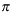Function Categories

# Mathematical Functions

The mathematical functions include a large proportion of the floating-point math functions usually provided with traditional UNIX C compilers. The header file `<math.h>` should be included when using most of these functions. See the individual function descriptions to determine whether the header file is required for that function.

The library also provides the standard header file `<float.h>` , which provides additional information about floating-point arithmetic. The contents of this header file are listed here:

```#define FLT_RADIX 16          /* hardware float radix               */
#define FLT_ROUNDS 0          /* float addition does not round.     */

#define FLT_MANT_DIG 6        /* hex digits in float mantissa       */
#define DBL_MANT_DIG 14       /* hex digits in double mantissa      */
#define LDBL_MANT_DIG 14      /* hex digits in long double mantissa */

#define FLT_DIG 6             /* float decimal precision            */
#define DBL_DIG 16            /* double decimal precision           */
#define LDBL_DIG 16           /* long double decimal precision      */

#define FLT_MIN_EXP -64       /* minimum exponent of 16 for float   */
#define DBL_MIN_EXP -64       /* minimum exponent of 16 for double  */
#define LDBL_MIN_EXP -64      /* minimum exponent of 16 for long    */
/* double                             */

#define FLT_MIN_10_EXP -78    /* minimum float power of 10          */
#define DBL_MIN_10_EXP -78    /* minimum double power of 10         */
#define LDBL_MIN_10_EXP -78   /* minimum long double power of 10    */

#define FLT_MAX_EXP 63        /* maximum exponent of 16 for float   */
#define DBL_MAX_EXP 63        /* maximum exponent of 16 for double  */
#define LDBL_MAX_EXP 63       /* maximum exponent of 16 for long    */
/* double                             */
#define FLT_MAX_10_EXP 75     /* maximum float power of 10          */
#define DBL_MAX_10_EXP 75     /* maximum double power of 10         */
#define LDBL_MAX_10_EXP 75    /* maximum long double power of 10    */

#define FLT_MAX .7237005e76F              /* maximum float          */
#define DBL_MAX .72370055773322621e76     /* maximum double         */
#define LDBL_MAX .72370055773322621e76L   /* maximum long double    */

/* smallest float x such that 1.0 + x != 1.0                     */
#define FLT_EPSILON .9536743e-6F

/* smallest double x such that 1.0 + x != 1.0                    */
#define DBL_EPSILON .22204460492503131e-15

/* smallest long double x such that 1.0 - x != 1.0               */
#define LDBL_EPSILON .22204460492503131e-15L

#define FLT_MIN .5397606e-78F                /* minimum float       */
#define DBL_MIN .53976053469340279e-78       /* minimum double      */
#define LDBL_MIN .53976053469340279e-78L     /* minimum long double */```

Additionally, the header file `<lcmath.h>` declares useful mathematical constants, as listed in Constant Values Declared in lcmath.h.

Constant Values Declared in lcmath.h
Constant Representation
M_PIM_PI_2/2
M_PI_4/4
M_1_PI 1/M_2_PI 2/M_E e
HUGE* largest possible double
TINY double closest to zero
LOGHUGE log(HUGE)
LOGTINY log(TINY)

In Constant Values Declared in lcmath.h, `math.h` defines the value `HUGE_VAL` , which is an ANSI-defined symbol with the same value.

The following are mathematical functions:
 `abs` integer conversion: absolute value `acos` compute the trigonometric arc cosine `asin` compute the trigonometric arc sine `atan` compute the trigonometric arc tangent `atan2` compute the trigonometric arc tangent of a quotient `ceil` round up a floating-point number `cos` compute the trigonometric cosine `cosh` compute the hyperbolic cosine `div` integer conversion: division `erf` compute the error function `erfc` compute the complementary error function `exp` compute the exponential function `fabs` floating-point conversion: absolute value `floor` round down a floating-point number `fmax` find the maximum of two doubles `fmin` find the minimum of two doubles `fmod` floating-point conversion: modules `frexp` floating-point conversion: fraction-exponent split `gamma` compute the logarithm of the gamma function `hypot` compute the hypotenuse function `j0` Bessel function of the first kind, order 0 `j1` Bessel function of the first kind, order 1 `jn` Bessel function of the first kind, order n `labs` integer conversion: absolute value `llabs` integer conversion: absolute value `ldexp` floating-point conversion: load exponent `_ldexp` fast implementation of `ldexp` `ldiv` integer conversion: division `lldiv` integer conversion: division `llmax` find the maximum of two integers `llmin` find the minimum of two integers `log` compute the natural logarithm `log10` compute the common logarithm `_matherr` handle math function error `max` find the maximum of two integers `min` find the minimum of two integers `modf` floating-point conversion: fraction-integer split `pow` compute the value of the power function `rand` simple random number generation `sin` compute the trigonometric sine `sinh` compute the hyperbolic sine `sqrt` compute the square root `srand` simple random number generation `tan` compute the trigonometric tangent `tanh` compute the hyperbolic tangent `y0` Bessel function of the second kind, order 0 `y1` Bessel function of the second kind, order 1 `yn` Bessel function of the second kind, order n.